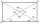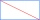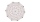# Right triangle + rectangle - math problems

#### Number of problems found: 71

• A rectangle 5A rectangle has sides of 10 cm and 14 cm. Calculate the angle between a diagonal and alongside.
• JoanneJoanne and Roger are planting a rectangular garden. The garden is 8 1/2 ft by 13 ft. They want to use half of the garden for cucumbers and half of the garden for tomatoes. They decide to separate the garden into two right triangles. What is the area of th
• Triangular landJana has a rectangular garden measuring 30 meters by 72 meters that she wants to split diagonally from corner to corner using a fence. How long does her fence need to be?
• Angle of diagonalsCalculate the perimeter and the area of a rectangle if its diagonal is 14 cm and the diagonals form an angle of 130°.
• Side and diagonalFind the circumference and the area of the rectangle if given: side a = 8 cm diagonal u = 10 cm.
• Diagonals of a prismThe base of the square prism is a rectangle with dimensions of 3 dm and 4 dm. The height of the prism is 1 m. Find out the angle between the body diagonal with the diagonal of the base.
• Metal washersMetal washers with a diameter of 80 mm are cut from a strip of steel sheet with a width of 10 cm and a length of 2 m. Calculate the percentage of material waste if no material is lost when two adjacent circles meet.
• GardensThe area of the square garden is 3/4 of the area of the triangular garden with sides of 80 m, 50 m, 50 m. How many meters of the fence do we need to fence a square garden?A quadrilateral pyramid, which has a rectangular base with dimensions of 24 cm, 13 cm. The height of the pyramid is 18cm. Calculate 1/the area of the base 2/casing area 3/pyramid surface 4/volume of the pyramid
• Perimeter and diagonalThe perimeter of the rectangle is 82 m, the length of its diagonal is 29 m. Find the dimensions of the rectangle.Calculate the surface of a quadrilateral pyramid, which has a rectangular base with dimensions a = 8 cm, b = 6 cm and height H = 10 cm.
• Triangular prismCalculate the surface of a regular triangular prism, the edges of the base are 6 cm long and the height of the prism is 15 cm.
• Height of the cuboidCuboid with a rectangular base, measuring 3 cm and 4 cm diagonal has a body 13 centimeters long. What is the height of the cuboid?
• Tetrahedral pyramid 8Let’s all side edges of the tetrahedral pyramid ABCDV be equally long and its base let’s be a rectangle. Determine its volume if you know the deviations A=40° B=70° of the planes of adjacent sidewalls and the plane of the base and the height h=16 of the p
• The pyramid 4sThe pyramid with a rectangular base measuring 6 dm and 8 dm has a side edge of length 13 dm. Calculate the surface area and volume of this pyramid.
• Rectangular base pyramidCalculate an area of the shell of the pyramid with a rectangular base of 2.8 m and 1.4 m and height 2.5 meters.The quadrilateral pyramid has a rectangular base of 24 cm x 3.2dm and a body height of 0.4m. Calculate its volume and surface area.
• A rectangle 2A rectangle has a diagonal length of 74cm. Its side lengths are in ratio 5:3. Find its side lengths.
• Faces diagonalsIf a cuboid's diagonals are x, y, and z (wall diagonals or three faces), then find the cuboid volume. Solve for x=1.3, y=1, z=1.2
• Wall and body diagonalsCalculate the lengths of the wall and body diagonals of the cuboid with edge dimensions of 0.5 m, 1 m, and 2 m

Do you have an exciting math question or word problem that you can't solve? Ask a question or post a math problem, and we can try to solve it.

We will send a solution to your e-mail address. Solved examples are also published here. Please enter the e-mail correctly and check whether you don't have a full mailbox.